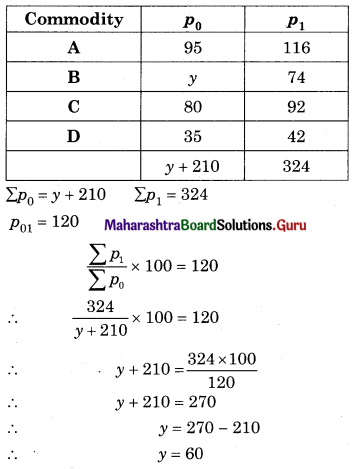Balbharati Maharashtra State Board 12th Commerce Maths Solution Book Pdf Chapter 5 Index Numbers Ex 5.1 Questions and Answers.

## Maharashtra State Board 12th Commerce Maths Solutions Chapter 5 Index Numbers Ex 5.1

Find the Price Index Number using the Simple Aggregate Method in each of the following examples.

Question 1.
Use 1995 as the base year in the following problem.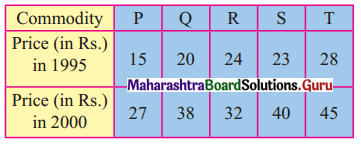Solution: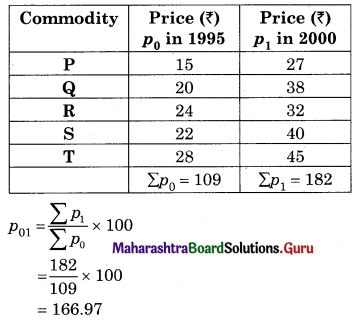Question 2.
Use 1995 as the base year in the following problem.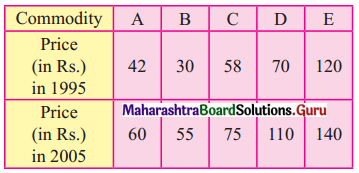Solution: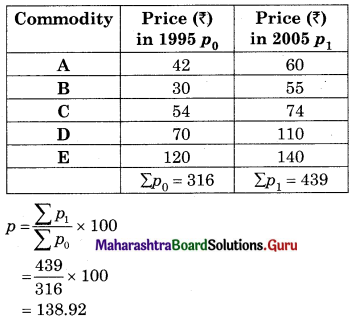Question 3.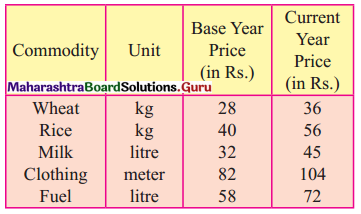Solution: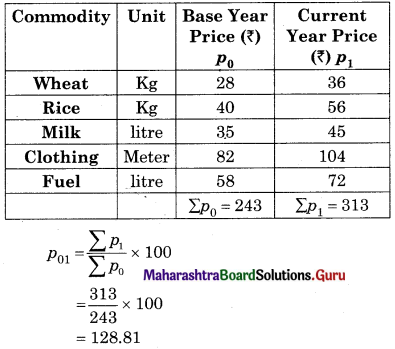Question 4.
Use 2000 as the base year in the following problem.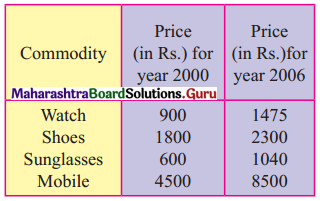Solution: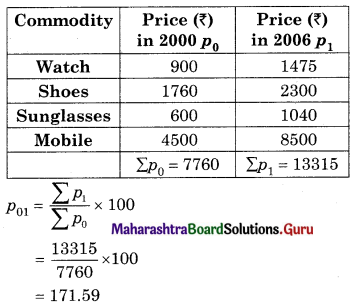Question 5.
Use 1990 as the base year in the following problem.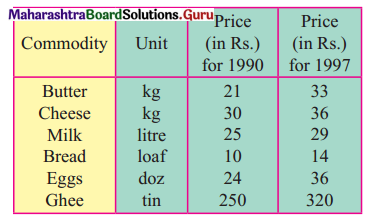Solution: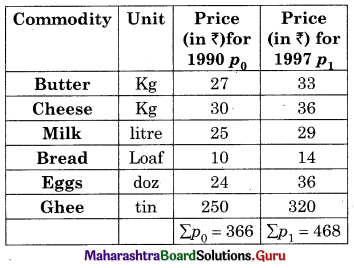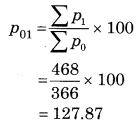Question 6.
Assume 2000 to be a base year in the following problem.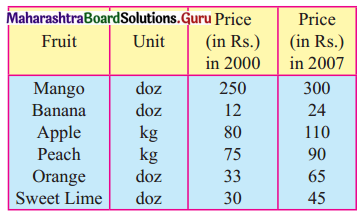Solution: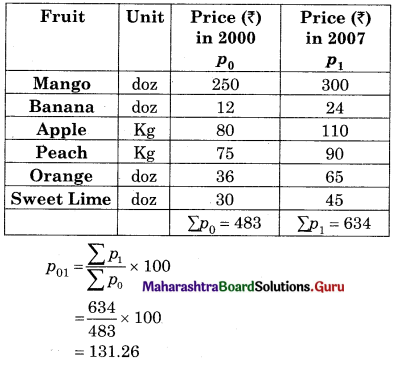Question 7.
Use 2005 as a year in the following problem.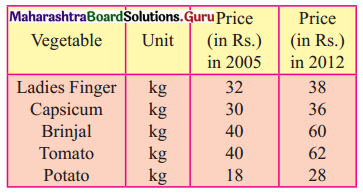Solution: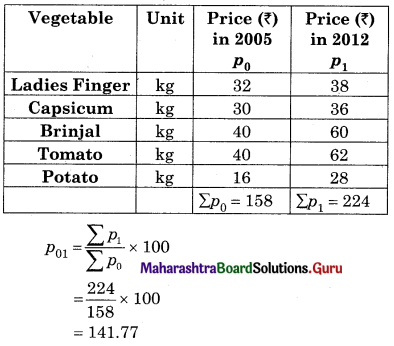Find the Quantity Index Number using the Simple Aggregate Method in each of the following examples.

Question 8.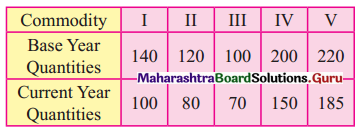Solution: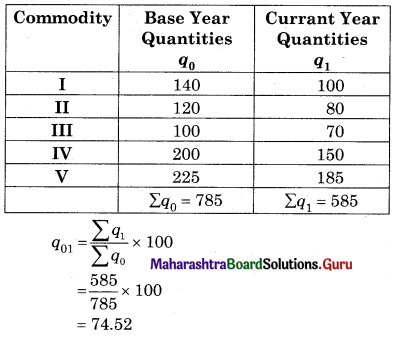Question 9.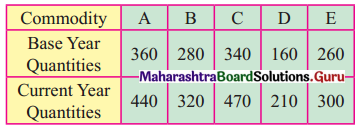Solution: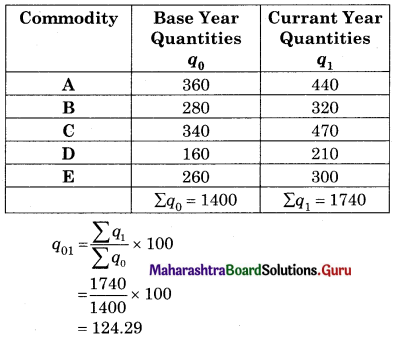Find the value Index Number using the Simple Aggregate Method in each of the following examples.

Question 10.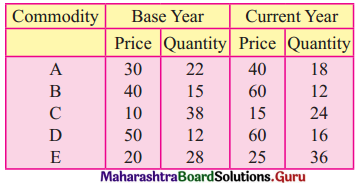Solution: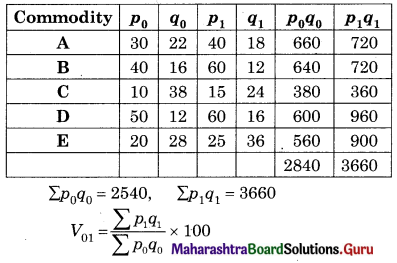= $$\frac{3660}{2840}$$ × 100
= 128.87

Question 11.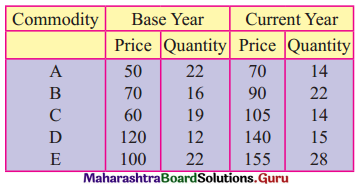Solution: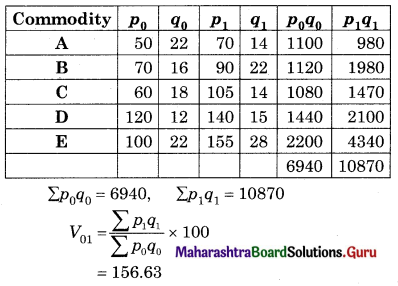Question 12.
Find x if the Price Index Number by Simple Aggregate Method is 125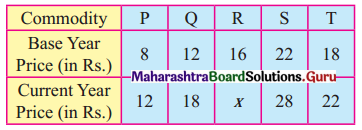Solution: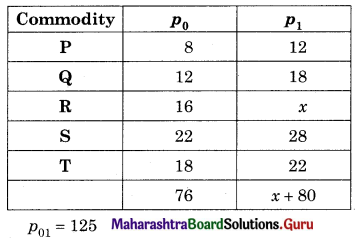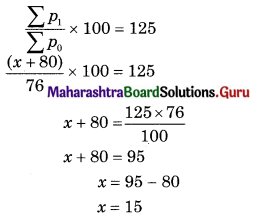Question 13.
Find y is the Price Index Number by Simple Aggregate Method is 120, taking 1995 as the base year.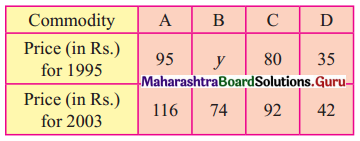Solution: